Quadratics appear all the time in algebra, and we know that x2 is positive (or zero) for every real x.  However, this still leaves the question of whether a quadratic function can return negative values.

So, can a quadratic be negative?  A quadratic can be negative (that is, have negative outputs or y values) for some or all of the x values in its domain.  A quadratic can have one, two, or three negative coefficients.  The zeros of a quadratic can also be negative.

Of course, there are some quadratics that are never negative.  For example, the quadratic f(x) = x2 + 1 has a minimum value of 1 on its domain (all real numbers).

Let’s get started.

## Can A Quadratic Be Negative?

A quadratic can be negative for some or all of the x values in its domain.  This will depend on the coefficients, but a quadratic with three negative coefficients is not necessarily always negative.

Remember that a quadratic function has the form:

• f(x) = ax2 + bx + c

where a, b, and c are real numbers and a is nonzero.

There are 3 basic cases for a quadratic and its y values (outputs):

• Always Negative – the y values of these quadratics are always negative (and possibly zero).  From a graphical perspective, the equation gives us a parabola that is always below (or on) the x axis.  The coefficient of x2 must be negative (that is, a < 0), but this alone does not guarantee that the quadratic will always be negative.
• Sometimes Negative – these quadratics have some negative y values, some positive y values, and some zero y values.  From a graphical perspective, the equation gives us a parabola that intersects the x-axis (sometimes it is below the x-axis, sometimes it is above the x-axis, and sometimes it is on the x-axis).
• Never Negative – the y values of these quadratics are never negative (they are always positive and possibly zero).  From a graphical perspective, the equation gives us a parabola that is always above the x axis.  The coefficient of x2 must be positive (that is, a > 0), but this alone does not guarantee that the quadratic will always be positive.

Let’s look at an example of each one of these cases.

### A Quadratic That Is Always Negative (Always Below The X-axis)

Consider the quadratic function f(x) = -x2 – 1.

This function will always be negative.  The reasoning is as follows:

• x2 >= 0  [for real x values]
• x2 + 1 >= 1  [add 1 to both sides of the equation above]
• -(x2 + 1) <= -1  [multiply both sides by -1, which means we must reverse the inequality symbol]
• -x2 – 1 <= -1  [distribute the -1 through parentheses]
• f(x) <= -1  [definition of f(x)]

Since f(x) is always less than or equal to 1, then the y-values of this quadratic are always negative.

So, this quadratic is always negative (below the x-axis).

You can see the graph of this function below.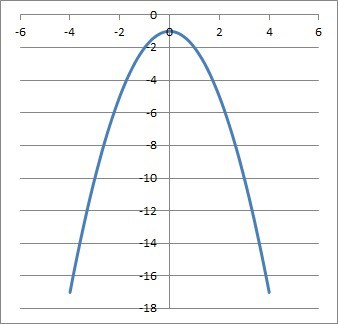The graph of the quadratic function f(x) = -x2 – 1 is a parabola that is always negative (below the x axis).

### A Quadratic That Is Sometimes Negative (Both Below & Above The X-axis)

Consider the quadratic function f(x) = x2 – 1.

This function will sometimes be negative, sometimes positive, and sometimes zero.  The reasoning is as follows:

f(2) = 22 – 1 = 4 – 1 = 3 > 0

f(1) = 12 – 1 = 1 – 1 = 0

f(0) = 02– 1 = 0 – 1 = -1 < 0

Since we can find three different x values to give us a positive, negative, and zero y values, this means that the function f(x) is sometimes negative, sometimes positive, and sometimes zero.

You can see the graph of this function below.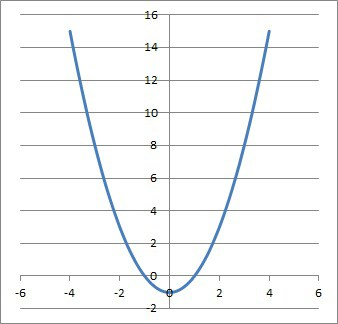The graph of the quadratic function f(x) = x2 – 1 is a parabola that is sometimes positive (above the x axis), sometimes negative (below the x axis), and sometimes zero (intersecting the x axis).

### A Quadratic That Is Never Negative (Always Above The X-axis)

Consider the quadratic function f(x) = x2 + 1.

This function will always be positive.  The reasoning is as follows:

• x2 >= 0  [for real x values]
• x2 + 1 >= 1  [add 1 to both sides of the equation above]
• f(x) >= 1  [definition of f(x)]

Since f(x) is always greater than or equal to 1, then the y-values of this quadratic are always positive.

So, this quadratic is always positive (above the x-axis) and never negative.

You can see the graph of this function below.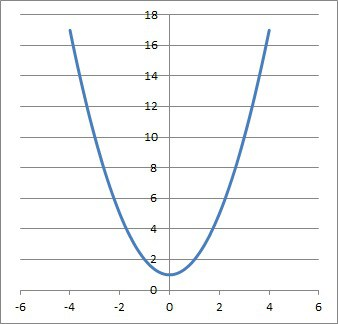The graph of the quadratic function f(x) = x2 + 1 is a parabola that is always positive (above the x axis).

## Can A Quadratic Function Be A Straight Line?

A quadratic function cannot be a straight line.  It will always have the shape of a parabola when graphed.

The reason comes from the definition of a quadratic function:

f(x) = ax2 + bx + c

where a, b, and c are real numbers and a is nonzero.

The key is the last part: a is nonzero.  If a = 0, then we would no longer have a quadratic function, but rather a linear function f(x) = bx + c.

## Can A Quadratic Function Be Periodic?

A quadratic function cannot be periodic.  A periodic function repeats y values at regular intervals, but a quadratic does not do this.

You can see the graph of a periodic function (sinusoidal) pictured in the graph below.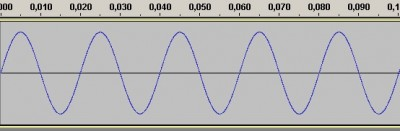The graph of a sine wave, which is periodic (y values in the range of the function repeat infinitely many times over the domain of all real numbers).

This function repeats the same y values infinitely many times over the range of the function (all real numbers).

However, a quadratic can repeat a y value at most once.  We can see this in the graph below by imagining any horizontal line, which intersects the function at most twice:

The reason is that for any y-value we can imagine (call it D), there are at most two possible real solutions to the equation f(x) = D or ax2 + bx + c = D.

## Can A Quadratic Function Have 3 Zeros?

A quadratic function cannot have 3 zeros.  A quadratic function can have at most 2 real zeros.

The cases for the solutions (zeros) of a quadratic are as follows:

• Two Real Roots – this happens when the discriminant b2 – 4ac > 0 (that is, when the expression under the radical in the quadratic formula is positive).
• One Real Root – this happens when the discriminant b2 – 4ac = 0.
• No Real Roots – this happens when the discriminant b2 – 4ac < 0.

We can also see this from the graph of a quadratic: a horizontal line will intersect a parabola at most two times.  So, the horizontal line y = 0 can only intersect a parabola twice, not three times.

Remember that the zeros (roots) of a quadratic can also be negative in some cases.

For example:

• f(x) = x2 + 3x + 2 factors as (x + 1)(x + 2), which has two negative zeros: x = -1 and x = -2.
• f(x) = x2 + 2x + 1 factors as (x + 1)(x + 1), which has one negative zeros: x = -1 (a real repeated root).
• f(x) = x2 – 3x + 2 factors as (x – 1)(x – 2), which has two positive zeros: x = 1 and x = 2 (no negative zeros).

## Can A Quadratic Function Open Sideways?

A quadratic that opens sideways, such as x = y2, is not a function, since it fails the vertical line test.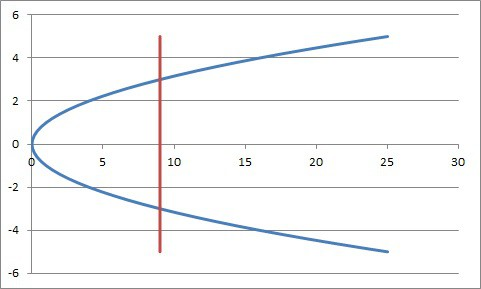The sideways quadratic x = y2 is a relation, but it is not a function, since it fails the vertical line test.

We can see this easily with the points (1, -1) and (1, 1), which are both on the graph of x = y2.  They both lie on the vertical line x = 1.

This means the vertical line x = 1 intersects the parabola x = y2 twice.  So, this relation fails the vertical line test.

## Can A Quadratic Function Have An Inverse?

A quadratic function cannot have an inverse if it is defined over the set of real numbers.

A function must be one-to-one to have an inverse.  However, a quadratic is not one-to-one on its domain of the entire set of real numbers (that is, every quadratic function fails the horizontal line test).

However, we can restrict the domain of a quadratic function to make it one-to-one.  In that case, it would have an inverse on the restricted domain.

To do this, we would need to restrict the quadratic function’s domain to x >= v, where v is the vertex of the parabola.  Remember that the x-coordinate of the vertex of a parabola is v = -b/2a.

We would also need to restrict the domain so that we only take positive square roots.

So, for the quadratic function f(x) = 3x2 + 24x + 48, we would need to restrict the domain to x >= v, where v = -b / 2a = -(24) / 2(3) = -24 / 6 = -4.

So, f(x) = 3x2 + 24x + 48 has an inverse if the domain is restricted to x >= -4.

To find its inverse, we let f(x) = y and solve for x:

• y = 3x2 + 24x + 48
• y = 3(x2 + 8x + 16)  [factor out a GCF of 3 on the right side]
• y = 3(x + 4)2  [factor the perfect square trinomial]
• y / 3 = (x + 4)2  [divide by 3 on both sides]
• (y / 3)1/2 = x + 4  [take the square root of both sides]
• (y / 3)1/2 – 4 = x  [subtract 4 from both sides]

Thus, the inverse is f-1(x) = (x / 3)1/2 – 4  (valid for x >= 0).

## Can A Quadratic Function Have A Local Maximum?

A quadratic function can have a local maximum, which is in fact its global or absolute maximum.

Any quadratic function with a negative x2 coefficient (that is, a < 0) will have a local maximum at its vertex.

We can see this from the graph of a parabola with a < 0:

We can also see this with calculus by taking derivatives.

If f(x) = ax2 + bx + c, then:

• The first derivative is f’(x) = 2ax + b, which is zero at x = -b / 2a (that is, the vertex is a critical point of the function).
• The second derivative is f’’(x) = 2a, which is always negative for a < 0.  This means the function is always concave down, and the vertex is the highest point, or a local maximum.

On the other hand, a parabola with a > 0 will always have a local minimum (which is also a global or absolute minimum) at the vertex.